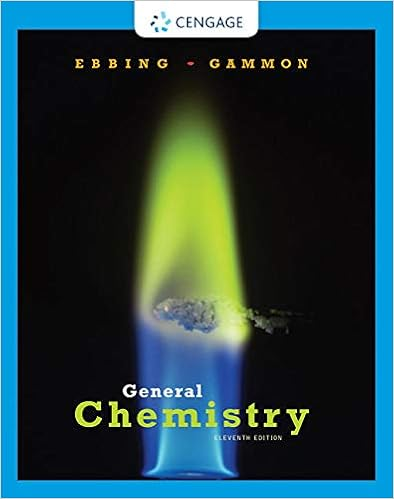# Based on your data what relationship exists between

• Lab Report
• 7
• 91% (89) 81 out of 89 people found this document helpful

This preview shows page 4 - 6 out of 7 pages.

##### We have textbook solutions for you!
The document you are viewing contains questions related to this textbook.The document you are viewing contains questions related to this textbook.
Chapter 5 / Exercise 5.5
General Chemistry
Ebbing/GammonExpert Verified
6. Based on your data, what relationship exists between the temperature and the volume of a gas(assuming a constant pressure)?
7. Look up a statement of Charles’ Law in your textbook. Do your results further prove this?
8. Using the spreadsheet program, fit the ideal gas data to a line or printout the graph and use a ruler todraw the best line through the data. The lowest possible temperature is reached when an ideal gas haszero volume. This temperature is the x-intercept for the plotted line.What is this temperature?
9. Now do the same analysis with the real gas data (N2). What temperature did you find?
10. Under these conditions, does N2 behave like an ideal gas?
5-4: Derivation of the Ideal Gas LawAn ideal gas is a hypothetical gas whose pressure, volume, and temperature follow the relationship PV =nRT. Ideal gases do not actually exist, although all real gases can behave like an ideal gas at certaintemperatures and pressures. All gases can be described to some extent using the Ideal Gas Law, and it isimportant in our understanding of how all gases behave. In this assignment, you will derive the Ideal GasLaw from experimental observations.The state of any gas can be described using the four variables: pressure (P), volume (V), temperature (T),and the number of moles of gas (n). Each experiment in Virtual ChemLab: Gases allows three of thesevariables (the independent variables) to be manipulated or changed and shows the effect on the remainingvariable (the dependent variable).1. Start Virtual ChemLab and select Ideal Gas Law from the list of assignments. The lab will open in theGases laboratory.2. Use the balloon experiment already setup in the laboratory to describe the relationship betweenpressure (P) and volume (V). Increase and decrease the pressure using the lever on the Pressure LCDcontroller to determine the effect on volume. What can you conclude about the effect of pressure on volume? Write a mathematical relationship
##### We have textbook solutions for you!
The document you are viewing contains questions related to this textbook.The document you are viewing contains questions related to this textbook.
Chapter 5 / Exercise 5.5
General Chemistry
Ebbing/GammonExpert Verified
3. Use this same experiment to describe the relationship between temperature (T) and volume byincreasing and decreasing the temperature. What can you conclude about the effect of temperature on volume? Write a mathematical relationship using the proportionality symbol ().
•••